Скачать презентацию Vectors and Coordinate Systems Chapter Goal To learn

• Количество слайдов: 62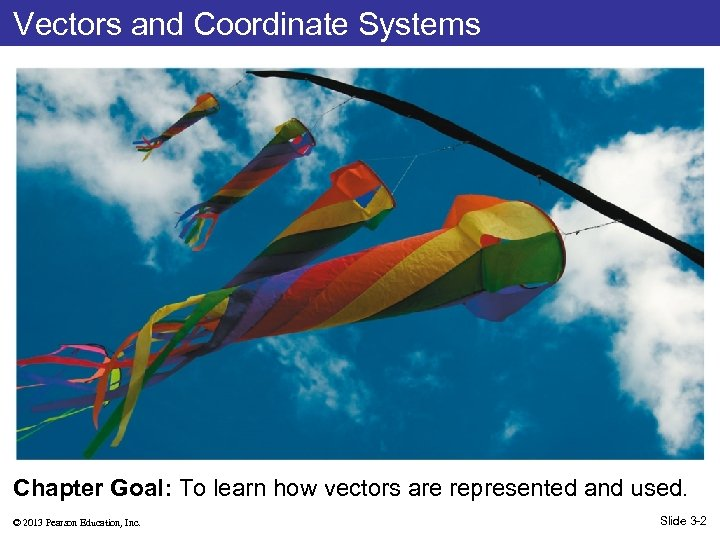Vectors and Coordinate Systems Chapter Goal: To learn how vectors are represented and used. © 2013 Pearson Education, Inc. Slide 3 -2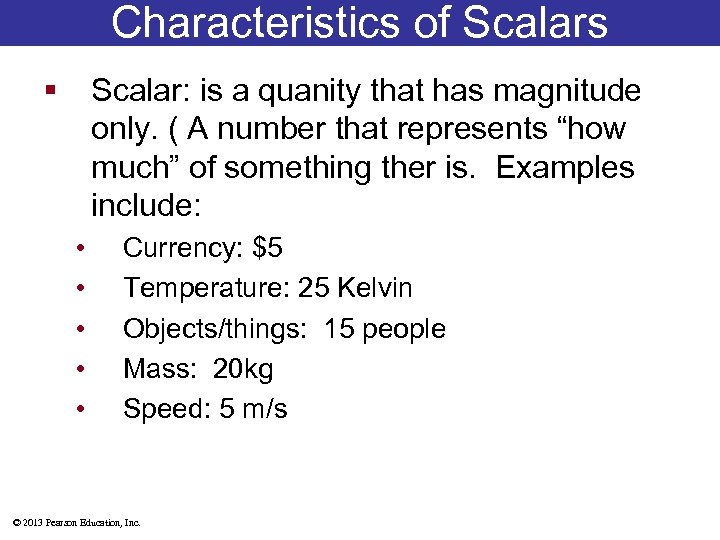Characteristics of Scalars § Scalar: is a quanity that has magnitude only. ( A number that represents “how much” of something ther is. Examples include: • • • Currency: \$5 Temperature: 25 Kelvin Objects/things: 15 people Mass: 20 kg Speed: 5 m/s © 2013 Pearson Education, Inc.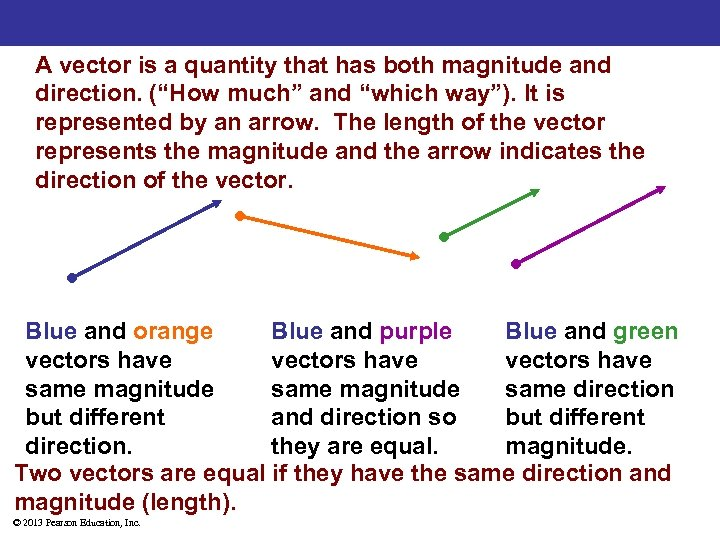A vector is a quantity that has both magnitude and direction. (“How much” and “which way”). It is represented by an arrow. The length of the vector represents the magnitude and the arrow indicates the direction of the vector. Blue and orange Blue and purple Blue and green vectors have same magnitude same direction but different and direction so but different direction. they are equal. magnitude. Two vectors are equal if they have the same direction and magnitude (length). © 2013 Pearson Education, Inc.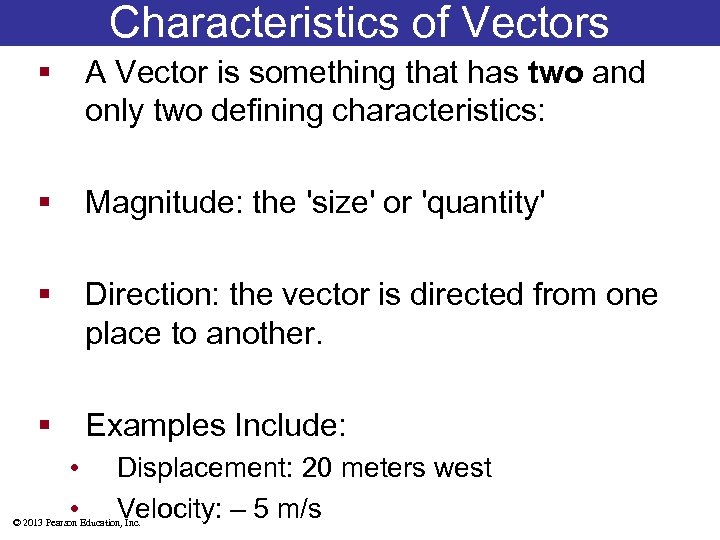Characteristics of Vectors § A Vector is something that has two and only two defining characteristics: § Magnitude: the 'size' or 'quantity' § Direction: the vector is directed from one place to another. § Examples Include: • • Displacement: 20 meters west Velocity: – 5 m/s © 2013 Pearson Education, Inc.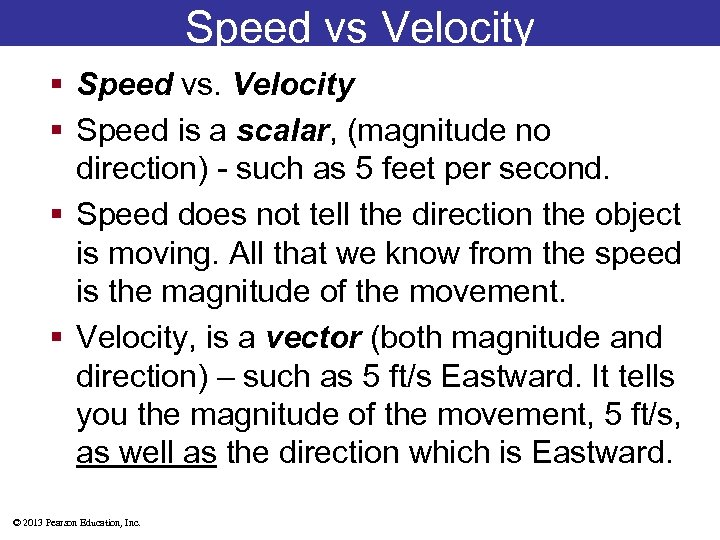Speed vs Velocity § Speed vs. Velocity § Speed is a scalar, (magnitude no direction) - such as 5 feet per second. § Speed does not tell the direction the object is moving. All that we know from the speed is the magnitude of the movement. § Velocity, is a vector (both magnitude and direction) – such as 5 ft/s Eastward. It tells you the magnitude of the movement, 5 ft/s, as well as the direction which is Eastward. © 2013 Pearson Education, Inc.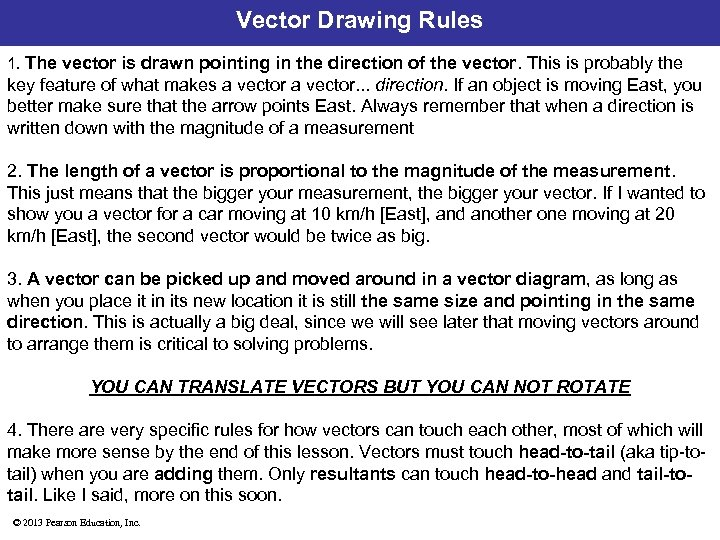Vector Drawing Rules 1. The vector is drawn pointing in the direction of the vector. This is probably the key feature of what makes a vector. . . direction. If an object is moving East, you better make sure that the arrow points East. Always remember that when a direction is written down with the magnitude of a measurement 2. The length of a vector is proportional to the magnitude of the measurement. This just means that the bigger your measurement, the bigger your vector. If I wanted to show you a vector for a car moving at 10 km/h [East], and another one moving at 20 km/h [East], the second vector would be twice as big. 3. A vector can be picked up and moved around in a vector diagram, as long as when you place it in its new location it is still the same size and pointing in the same direction. This is actually a big deal, since we will see later that moving vectors around to arrange them is critical to solving problems. YOU CAN TRANSLATE VECTORS BUT YOU CAN NOT ROTATE 4. There are very specific rules for how vectors can touch each other, most of which will make more sense by the end of this lesson. Vectors must touch head-to-tail (aka tip-totail) when you are adding them. Only resultants can touch head-to-head and tail-totail. Like I said, more on this soon. © 2013 Pearson Education, Inc.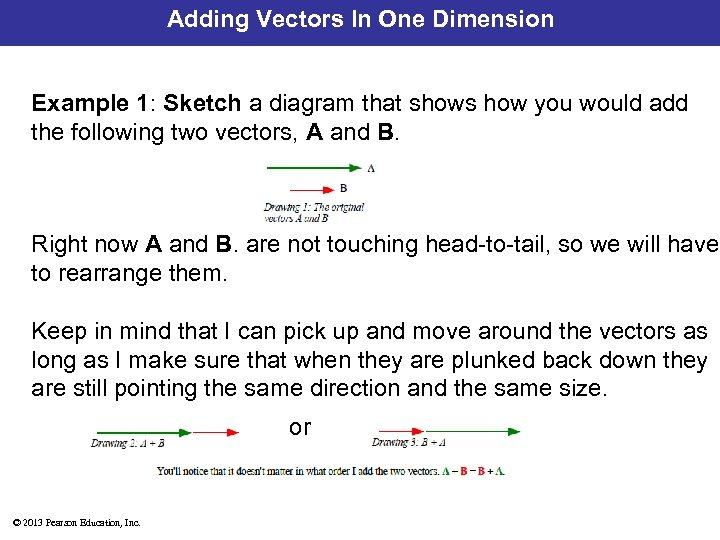Adding Vectors In One Dimension Example 1: Sketch a diagram that shows how you would add the following two vectors, A and B. Right now A and B. are not touching head-to-tail, so we will have to rearrange them. Keep in mind that I can pick up and move around the vectors as long as I make sure that when they are plunked back down they are still pointing the same direction and the same size. or © 2013 Pearson Education, Inc.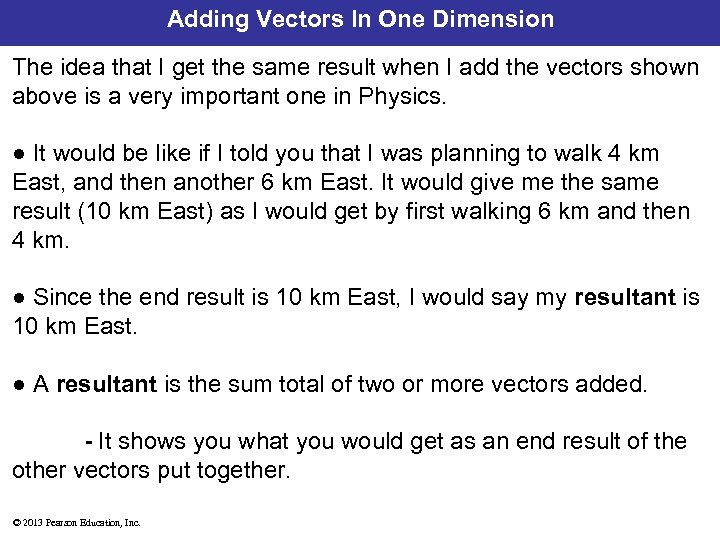Adding Vectors In One Dimension The idea that I get the same result when I add the vectors shown above is a very important one in Physics. ● It would be like if I told you that I was planning to walk 4 km East, and then another 6 km East. It would give me the same result (10 km East) as I would get by first walking 6 km and then 4 km. ● Since the end result is 10 km East, I would say my resultant is 10 km East. ● A resultant is the sum total of two or more vectors added. - It shows you what you would get as an end result of the other vectors put together. © 2013 Pearson Education, Inc.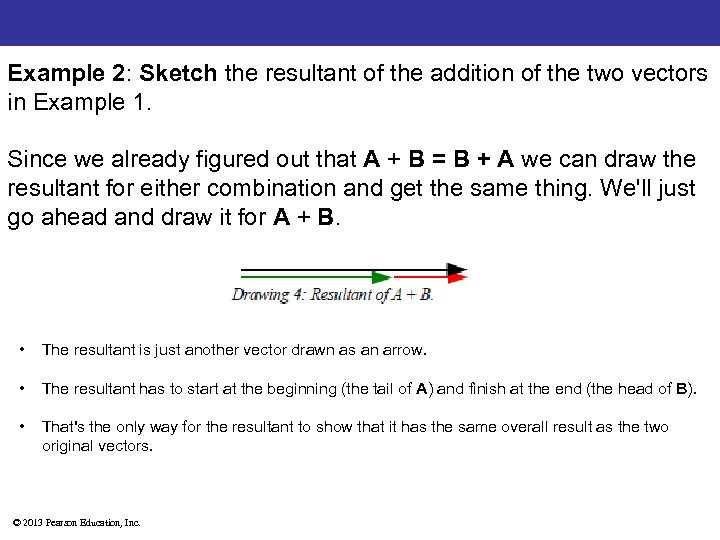Example 2: Sketch the resultant of the addition of the two vectors in Example 1. Since we already figured out that A + B = B + A we can draw the resultant for either combination and get the same thing. We'll just go ahead and draw it for A + B. • The resultant is just another vector drawn as an arrow. • The resultant has to start at the beginning (the tail of A) and finish at the end (the head of B). • That's the only way for the resultant to show that it has the same overall result as the two original vectors. © 2013 Pearson Education, Inc.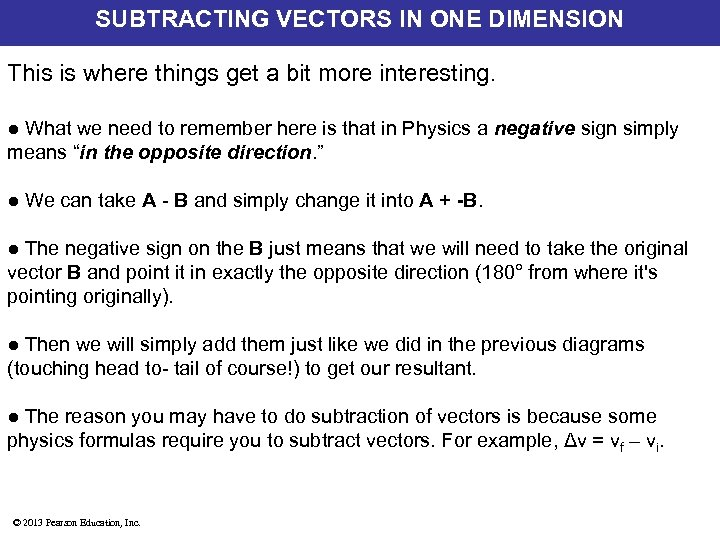SUBTRACTING VECTORS IN ONE DIMENSION This is where things get a bit more interesting. ● What we need to remember here is that in Physics a negative sign simply means “in the opposite direction. ” ● We can take A - B and simply change it into A + -B. ● The negative sign on the B just means that we will need to take the original vector B and point it in exactly the opposite direction (180° from where it's pointing originally). ● Then we will simply add them just like we did in the previous diagrams (touching head to- tail of course!) to get our resultant. ● The reason you may have to do subtraction of vectors is because some physics formulas require you to subtract vectors. For example, Δv = vf – vi. © 2013 Pearson Education, Inc.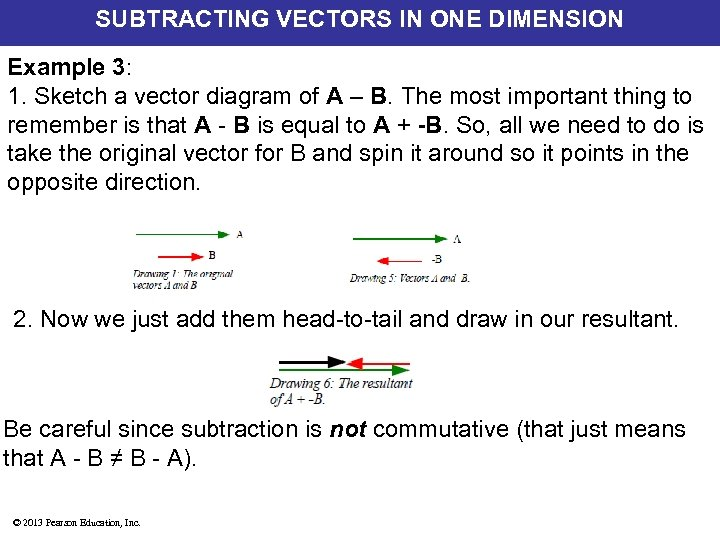SUBTRACTING VECTORS IN ONE DIMENSION Example 3: 1. Sketch a vector diagram of A – B. The most important thing to remember is that A - B is equal to A + -B. So, all we need to do is take the original vector for B and spin it around so it points in the opposite direction. 2. Now we just add them head-to-tail and draw in our resultant. Be careful since subtraction is not commutative (that just means that A - B ≠ B - A). © 2013 Pearson Education, Inc.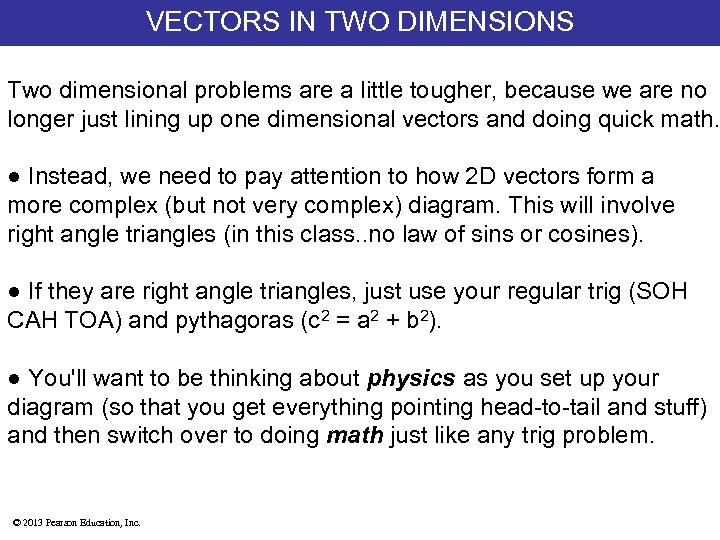VECTORS IN TWO DIMENSIONS Two dimensional problems are a little tougher, because we are no longer just lining up one dimensional vectors and doing quick math. ● Instead, we need to pay attention to how 2 D vectors form a more complex (but not very complex) diagram. This will involve right angle triangles (in this class. . no law of sins or cosines). ● If they are right angle triangles, just use your regular trig (SOH CAH TOA) and pythagoras (c 2 = a 2 + b 2). ● You'll want to be thinking about physics as you set up your diagram (so that you get everything pointing head-to-tail and stuff) and then switch over to doing math just like any trig problem. © 2013 Pearson Education, Inc.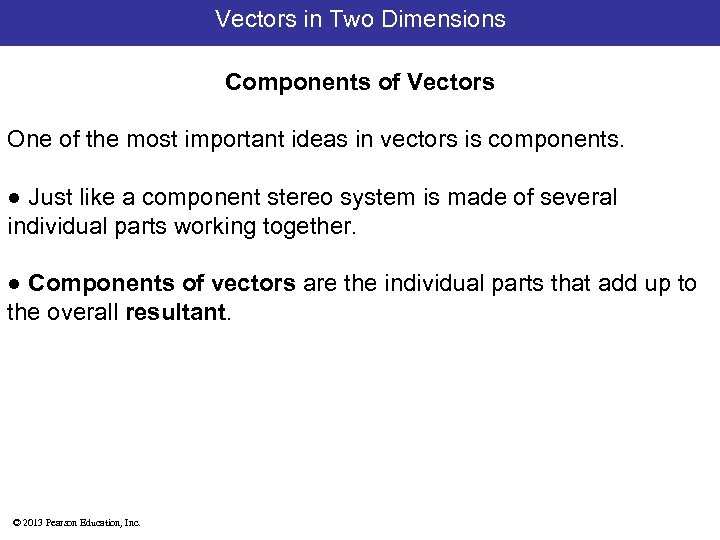Vectors in Two Dimensions Components of Vectors One of the most important ideas in vectors is components. ● Just like a component stereo system is made of several individual parts working together. ● Components of vectors are the individual parts that add up to the overall resultant. © 2013 Pearson Education, Inc.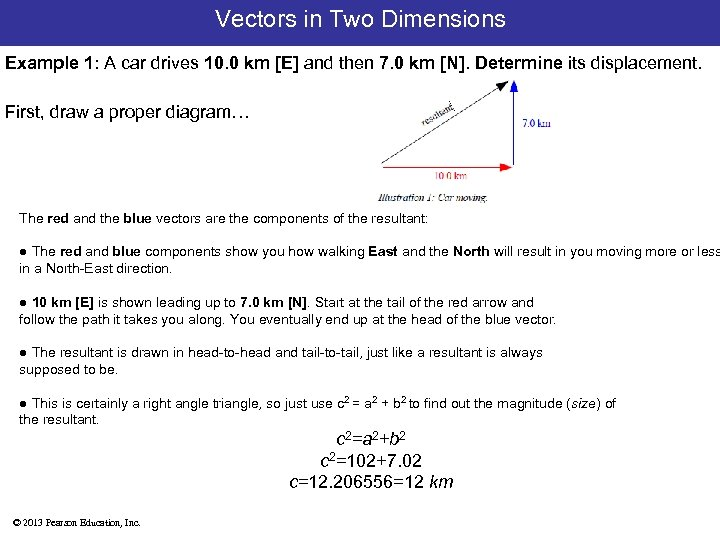Vectors in Two Dimensions Example 1: A car drives 10. 0 km [E] and then 7. 0 km [N]. Determine its displacement. First, draw a proper diagram. . . The red and the blue vectors are the components of the resultant: ● The red and blue components show you how walking East and the North will result in you moving more or less in a North-East direction. ● 10 km [E] is shown leading up to 7. 0 km [N]. Start at the tail of the red arrow and follow the path it takes you along. You eventually end up at the head of the blue vector. ● The resultant is drawn in head-to-head and tail-to-tail, just like a resultant is always supposed to be. ● This is certainly a right angle triangle, so just use c 2 = a 2 + b 2 to find out the magnitude (size) of the resultant. c 2=a 2+b 2 c 2=102+7. 02 c=12. 206556=12 km © 2013 Pearson Education, Inc.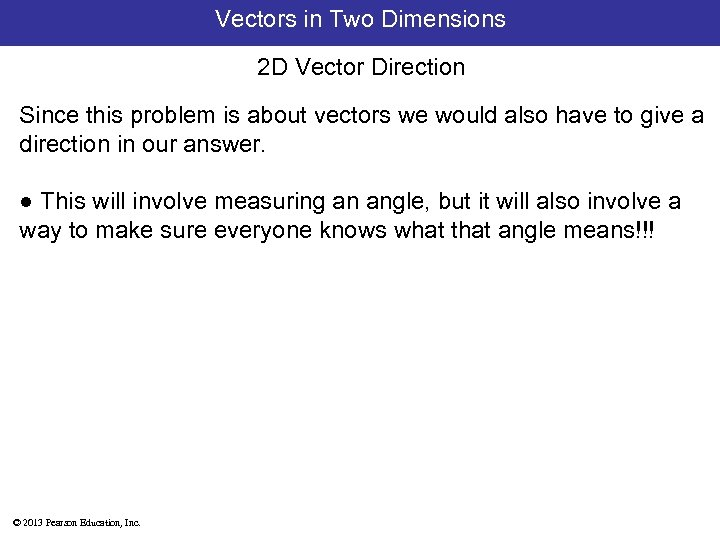Vectors in Two Dimensions 2 D Vector Direction Since this problem is about vectors we would also have to give a direction in our answer. ● This will involve measuring an angle, but it will also involve a way to make sure everyone knows what that angle means!!! © 2013 Pearson Education, Inc.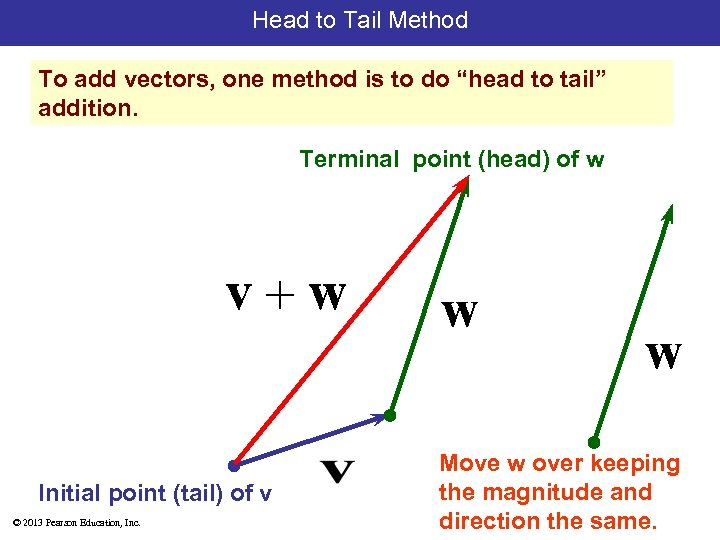Head to Tail Method To add vectors, one method is to do “head to tail” addition. Terminal point (head) of w Initial point (tail) of v © 2013 Pearson Education, Inc. Move w over keeping the magnitude and direction the same.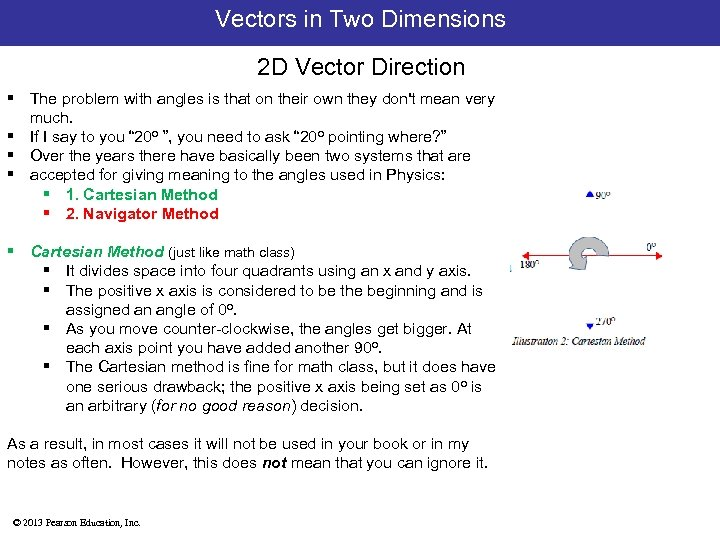Vectors in Two Dimensions 2 D Vector Direction § The problem with angles is that on their own they don't mean very much. § If I say to you “ 20 o ”, you need to ask “ 20 o pointing where? ” § Over the years there have basically been two systems that are § accepted for giving meaning to the angles used in Physics: § 1. Cartesian Method § 2. Navigator Method § Cartesian Method (just like math class) § It divides space into four quadrants using an x and y axis. § The positive x axis is considered to be the beginning and is assigned an angle of 0 o. § As you move counter-clockwise, the angles get bigger. At each axis point you have added another 90 o. § The Cartesian method is fine for math class, but it does have one serious drawback; the positive x axis being set as 0 o is an arbitrary (for no good reason) decision. As a result, in most cases it will not be used in your book or in my notes as often. However, this does not mean that you can ignore it. © 2013 Pearson Education, Inc.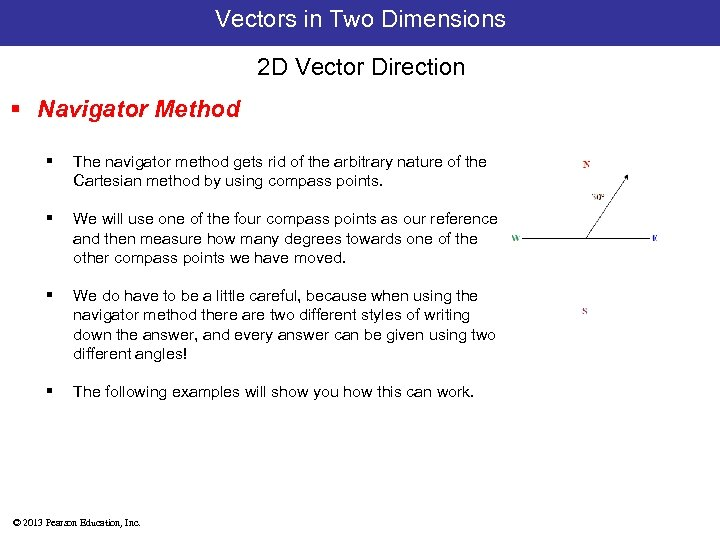Vectors in Two Dimensions 2 D Vector Direction § Navigator Method § The navigator method gets rid of the arbitrary nature of the Cartesian method by using compass points. § We will use one of the four compass points as our reference, and then measure how many degrees towards one of the other compass points we have moved. § We do have to be a little careful, because when using the navigator method there are two different styles of writing down the answer, and every answer can be given using two different angles! § The following examples will show you how this can work. © 2013 Pearson Education, Inc.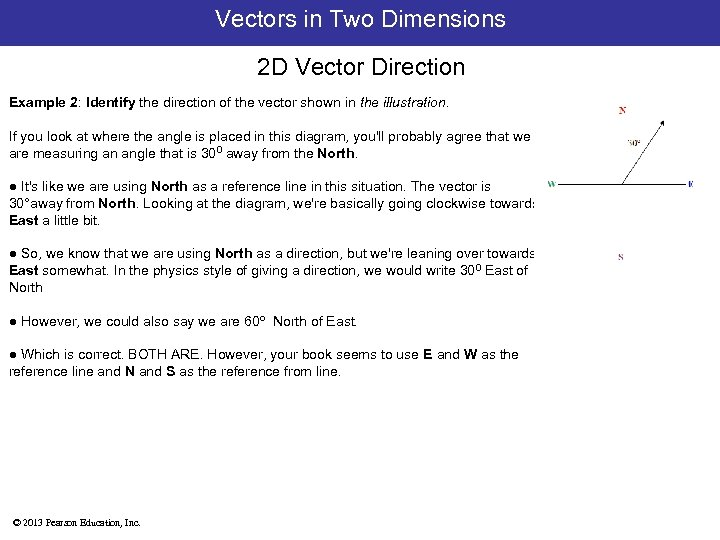Vectors in Two Dimensions 2 D Vector Direction Example 2: Identify the direction of the vector shown in the illustration. If you look at where the angle is placed in this diagram, you'll probably agree that we are measuring an angle that is 300 away from the North. ● It's like we are using North as a reference line in this situation. The vector is 30°away from North. Looking at the diagram, we're basically going clockwise towards East a little bit. ● So, we know that we are using North as a direction, but we're leaning over towards East somewhat. In the physics style of giving a direction, we would write 300 East of North ● However, we could also say we are 60 o North of East. ● Which is correct. BOTH ARE. However, your book seems to use E and W as the reference line and N and S as the reference from line. © 2013 Pearson Education, Inc.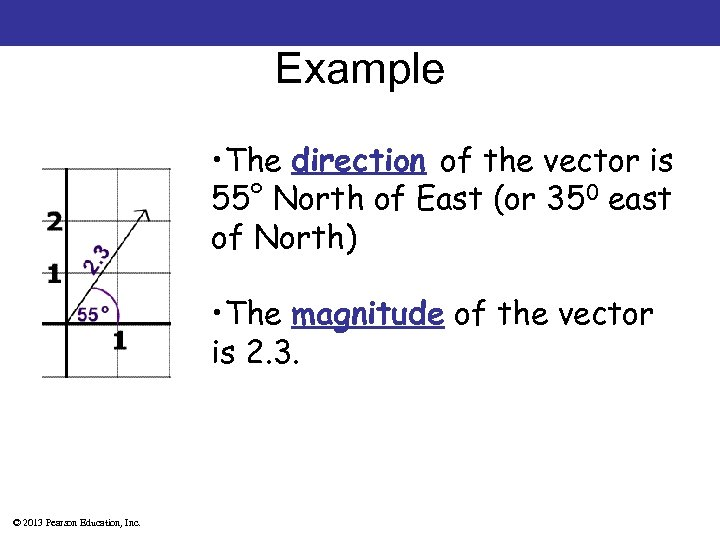Example • The direction of the vector is 55° North of East (or 350 east of North) • The magnitude of the vector is 2. 3. © 2013 Pearson Education, Inc.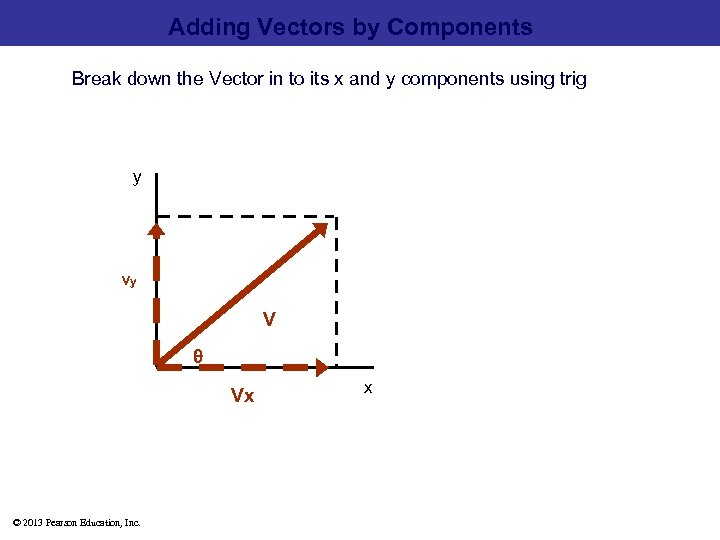Adding Vectors by Components Break down the Vector in to its x and y components using trig y Vy V θ Vx © 2013 Pearson Education, Inc. x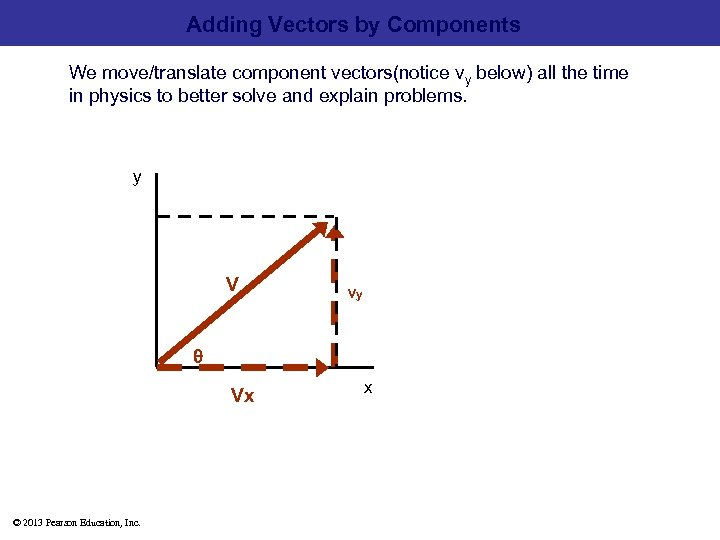Adding Vectors by Components We move/translate component vectors(notice vy below) all the time in physics to better solve and explain problems. y V Vy θ Vx © 2013 Pearson Education, Inc. x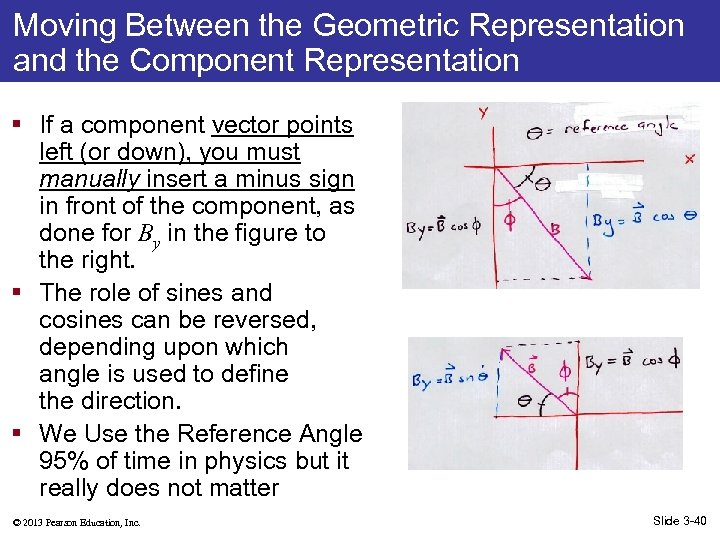Moving Between the Geometric Representation and the Component Representation § If a component vector points left (or down), you must manually insert a minus sign in front of the component, as done for By in the figure to the right. § The role of sines and cosines can be reversed, depending upon which angle is used to define the direction. § We Use the Reference Angle 95% of time in physics but it really does not matter © 2013 Pearson Education, Inc. Slide 3 -40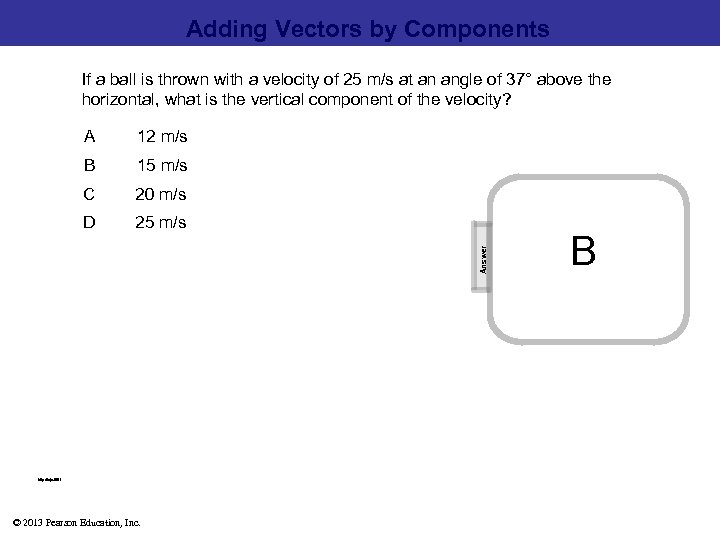Adding Vectors by Components If a ball is thrown with a velocity of 25 m/s at an angle of 37° above the horizontal, what is the vertical component of the velocity? 12 m/s B 15 m/s C 20 m/s D 25 m/s Answer A B [This object is a pull tab] http: //njc. tl/51 © 2013 Pearson Education, Inc.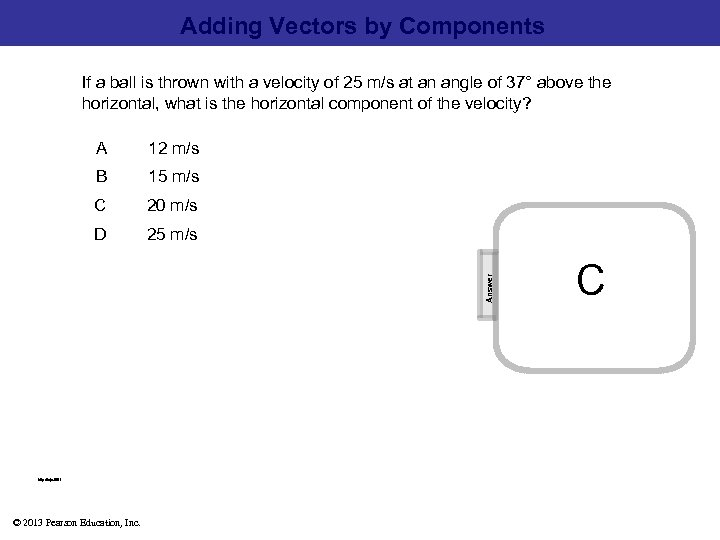Adding Vectors by Components If a ball is thrown with a velocity of 25 m/s at an angle of 37° above the horizontal, what is the horizontal component of the velocity? 12 m/s B 15 m/s C 20 m/s D 25 m/s Answer A C [This object is a pull tab] http: //njc. tl/51 © 2013 Pearson Education, Inc.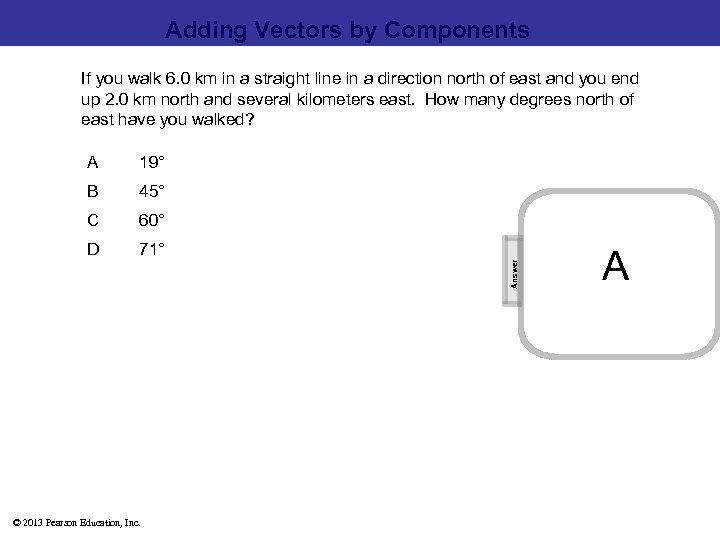Adding Vectors by Components If you walk 6. 0 km in a straight line in a direction north of east and you end up 2. 0 km north and several kilometers east. How many degrees north of east have you walked? 19° B 45° C 60° D 71° Answer A A [This object is a pull tab] © 2013 Pearson Education, Inc.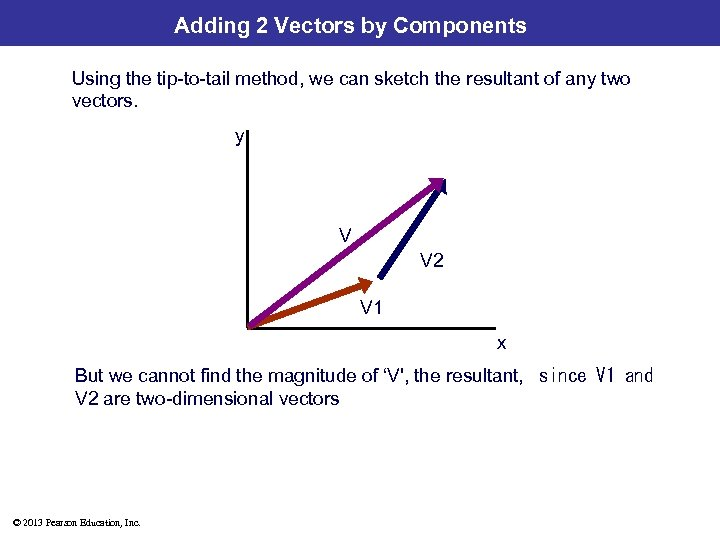Adding 2 Vectors by Components Using the tip-to-tail method, we can sketch the resultant of any two vectors. y V V 2 V 1 x But we cannot find the magnitude of ‘V', the resultant,  since V 1 and V 2 are two-dimensional vectors © 2013 Pearson Education, Inc.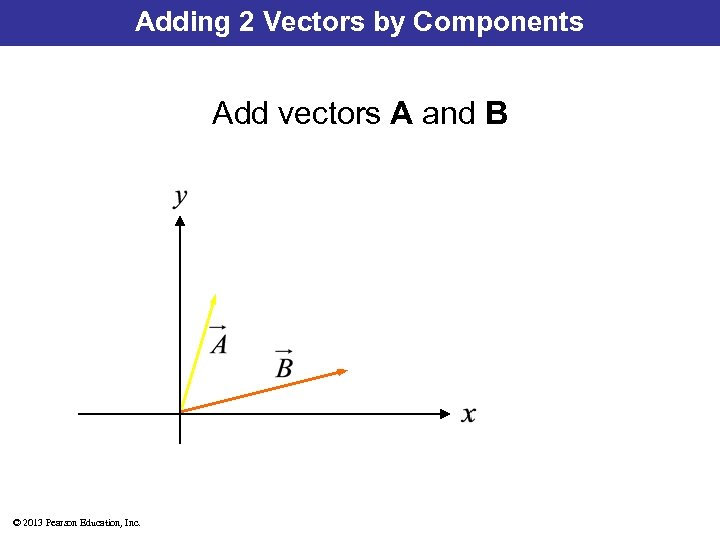Adding 2 Vectors by Components Add vectors A and B © 2013 Pearson Education, Inc.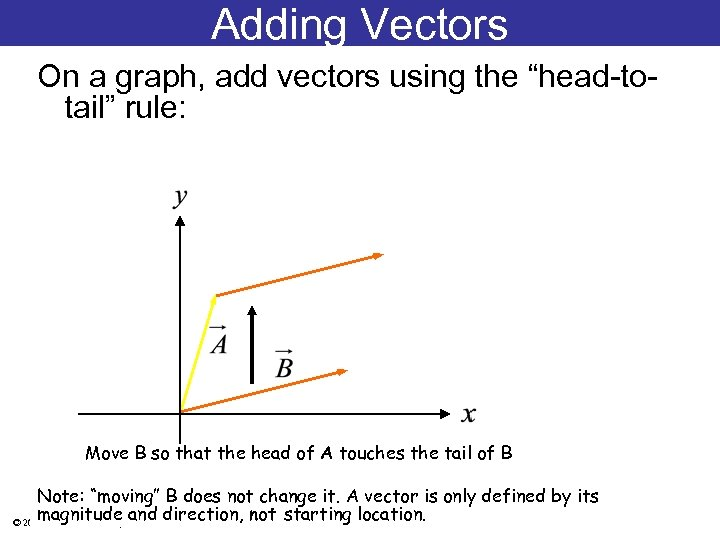Adding Vectors On a graph, add vectors using the “head-totail” rule: Move B so that the head of A touches the tail of B Note: “moving” B does not change it. A vector is only defined by its magnitude and direction, not starting location. © 2013 Pearson Education, Inc.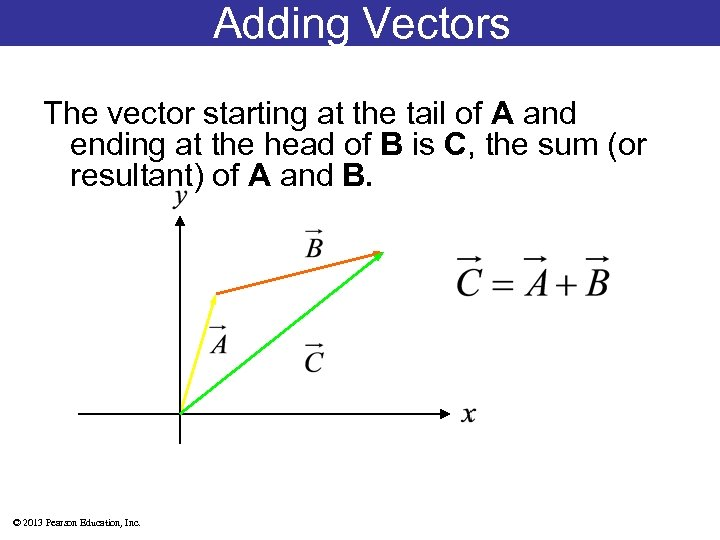Adding Vectors The vector starting at the tail of A and ending at the head of B is C, the sum (or resultant) of A and B. © 2013 Pearson Education, Inc.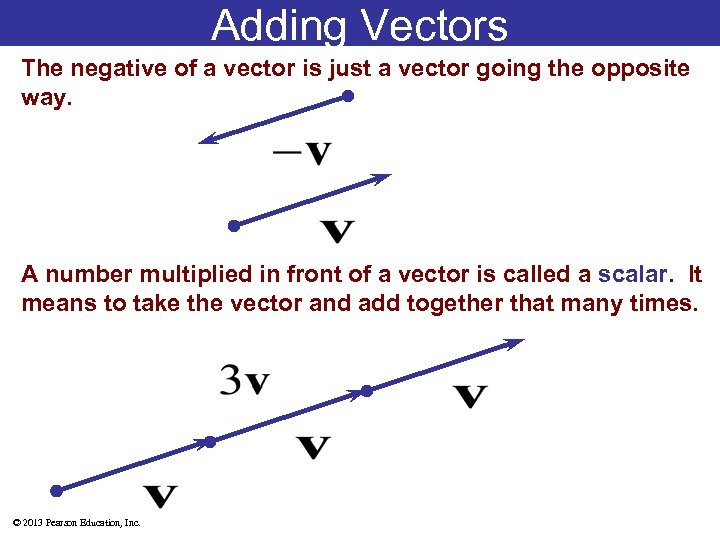Adding Vectors The negative of a vector is just a vector going the opposite way. A number multiplied in front of a vector is called a scalar. It means to take the vector and add together that many times. © 2013 Pearson Education, Inc.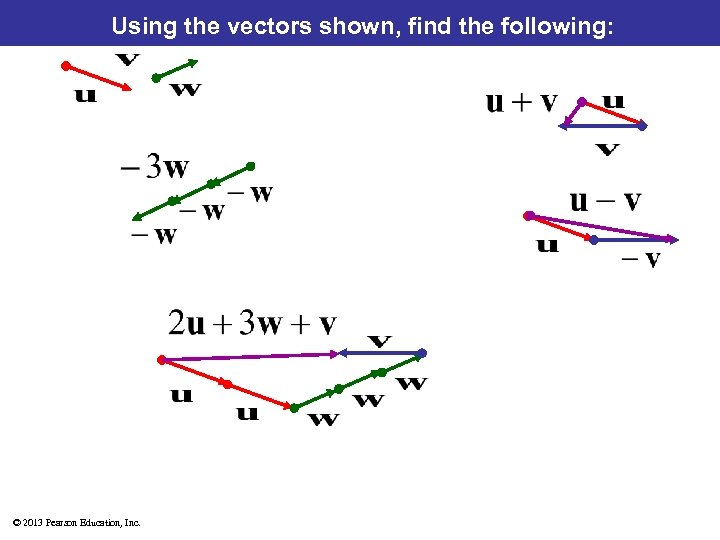Using the vectors shown, find the following: © 2013 Pearson Education, Inc.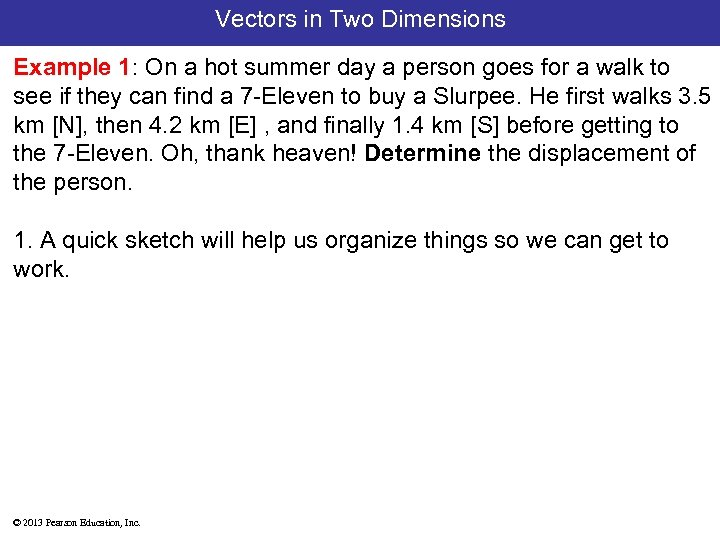Vectors in Two Dimensions Example 1: On a hot summer day a person goes for a walk to see if they can find a 7 -Eleven to buy a Slurpee. He first walks 3. 5 km [N], then 4. 2 km [E] , and finally 1. 4 km [S] before getting to the 7 -Eleven. Oh, thank heaven! Determine the displacement of the person. 1. A quick sketch will help us organize things so we can get to work. © 2013 Pearson Education, Inc.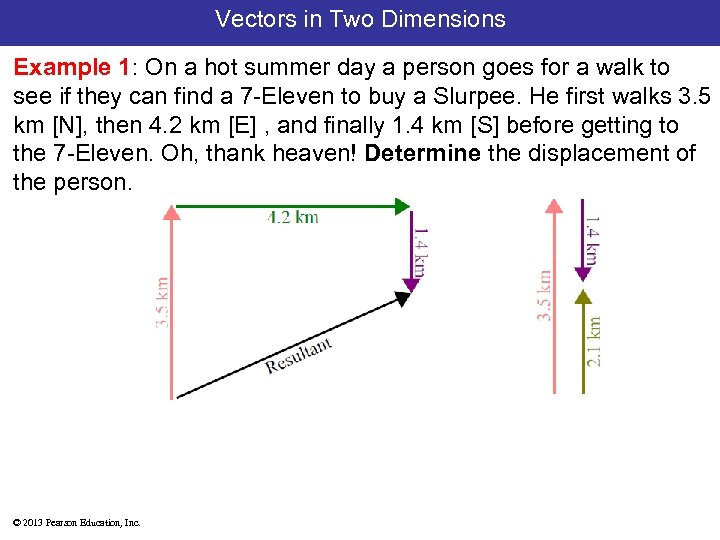Vectors in Two Dimensions Example 1: On a hot summer day a person goes for a walk to see if they can find a 7 -Eleven to buy a Slurpee. He first walks 3. 5 km [N], then 4. 2 km [E] , and finally 1. 4 km [S] before getting to the 7 -Eleven. Oh, thank heaven! Determine the displacement of the person. © 2013 Pearson Education, Inc.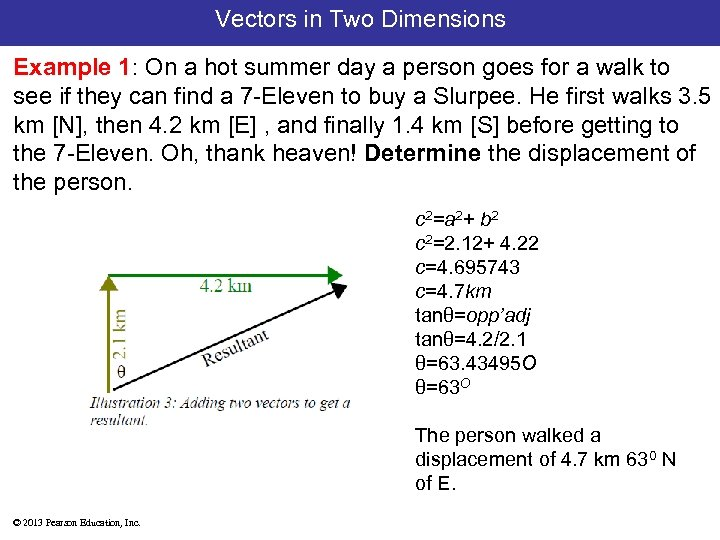Vectors in Two Dimensions Example 1: On a hot summer day a person goes for a walk to see if they can find a 7 -Eleven to buy a Slurpee. He first walks 3. 5 km [N], then 4. 2 km [E] , and finally 1. 4 km [S] before getting to the 7 -Eleven. Oh, thank heaven! Determine the displacement of the person. c 2=a 2+ b 2 c 2=2. 12+ 4. 22 c=4. 695743 c=4. 7 km tanθ=opp’adj tanθ=4. 2/2. 1 θ=63. 43495 O θ=63 O The person walked a displacement of 4. 7 km 630 N of E. © 2013 Pearson Education, Inc.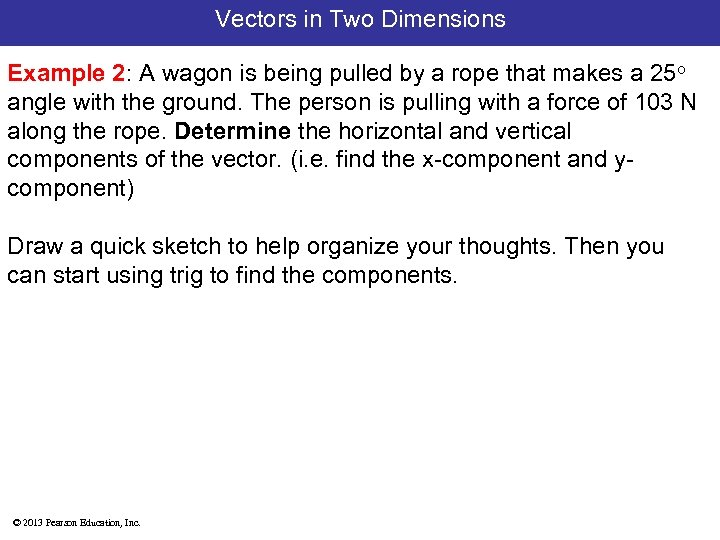Vectors in Two Dimensions Example 2: A wagon is being pulled by a rope that makes a 25 o angle with the ground. The person is pulling with a force of 103 N along the rope. Determine the horizontal and vertical components of the vector. (i. e. find the x-component and ycomponent) Draw a quick sketch to help organize your thoughts. Then you can start using trig to find the components. © 2013 Pearson Education, Inc.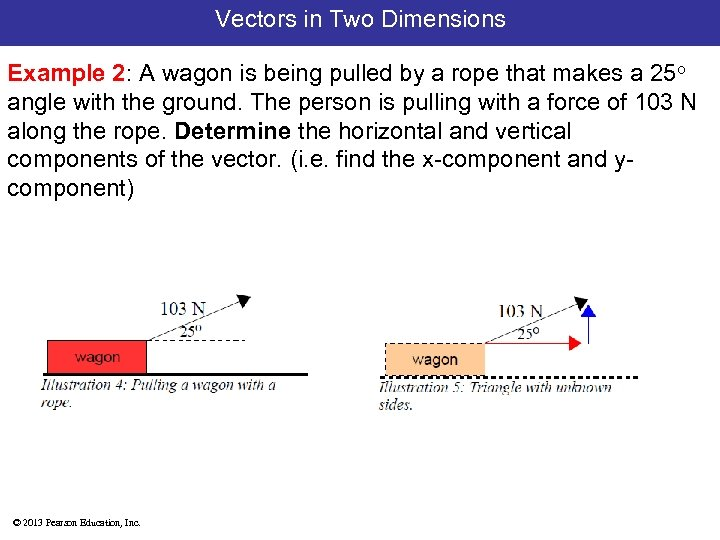Vectors in Two Dimensions Example 2: A wagon is being pulled by a rope that makes a 25 o angle with the ground. The person is pulling with a force of 103 N along the rope. Determine the horizontal and vertical components of the vector. (i. e. find the x-component and ycomponent) © 2013 Pearson Education, Inc.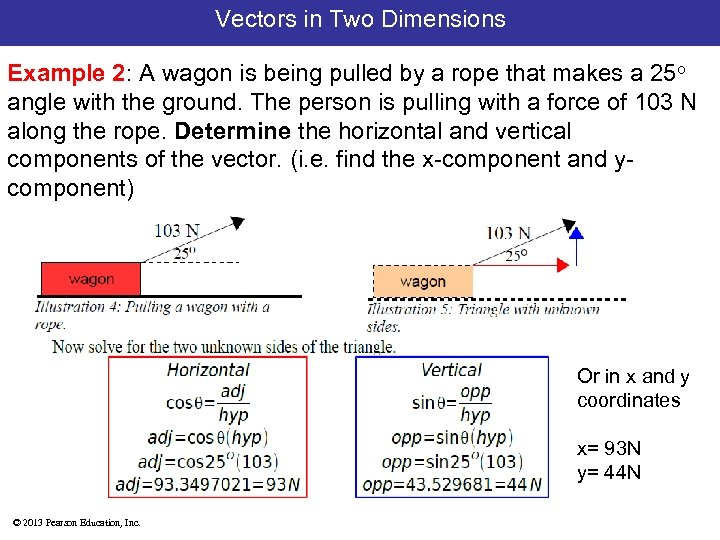Vectors in Two Dimensions Example 2: A wagon is being pulled by a rope that makes a 25 o angle with the ground. The person is pulling with a force of 103 N along the rope. Determine the horizontal and vertical components of the vector. (i. e. find the x-component and ycomponent) Or in x and y coordinates x= 93 N y= 44 N © 2013 Pearson Education, Inc.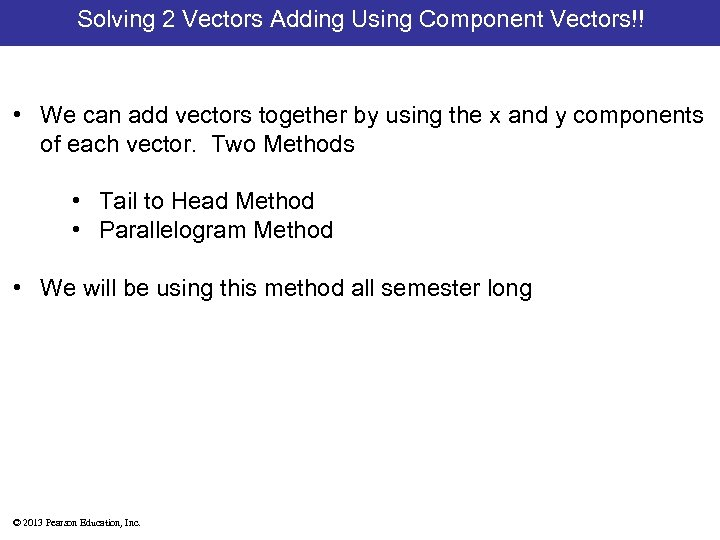Solving 2 Vectors Adding Using Component Vectors!! • We can add vectors together by using the x and y components of each vector. Two Methods • Tail to Head Method • Parallelogram Method • We will be using this method all semester long © 2013 Pearson Education, Inc.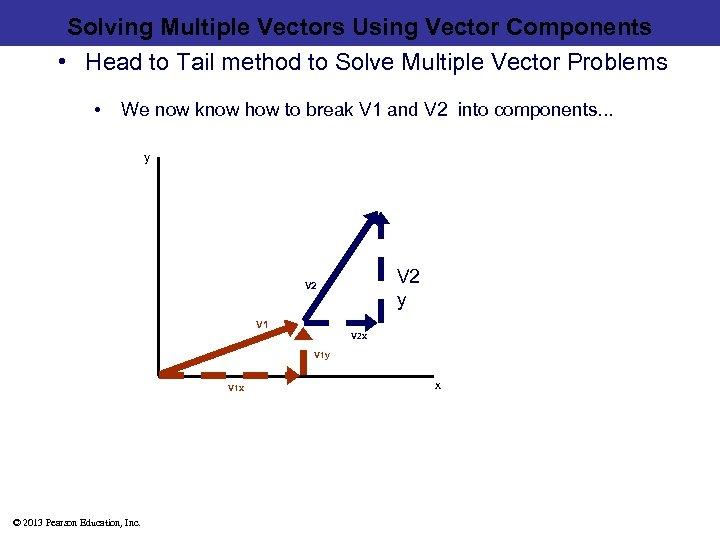Solving Multiple Vectors Using Vector Components • Head to Tail method to Solve Multiple Vector Problems • We now know how to break V 1 and V 2 into components. . . y V 2 V 1 V 2 x V 1 y V 1 x © 2013 Pearson Education, Inc. x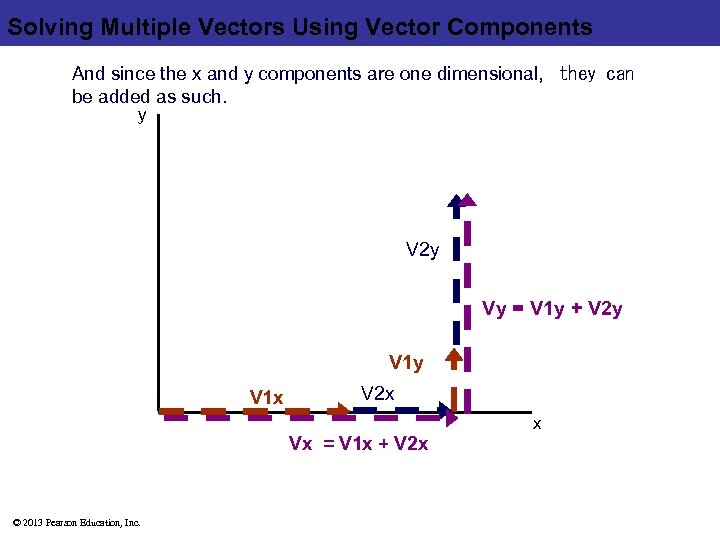Solving Multiple Vectors Using Vector Components And since the x and y components are one dimensional,  they can be added as such. y V 2 y Vy = V 1 y + V 2 y V 1 x V 2 x x Vx = V 1 x + V 2 x © 2013 Pearson Education, Inc.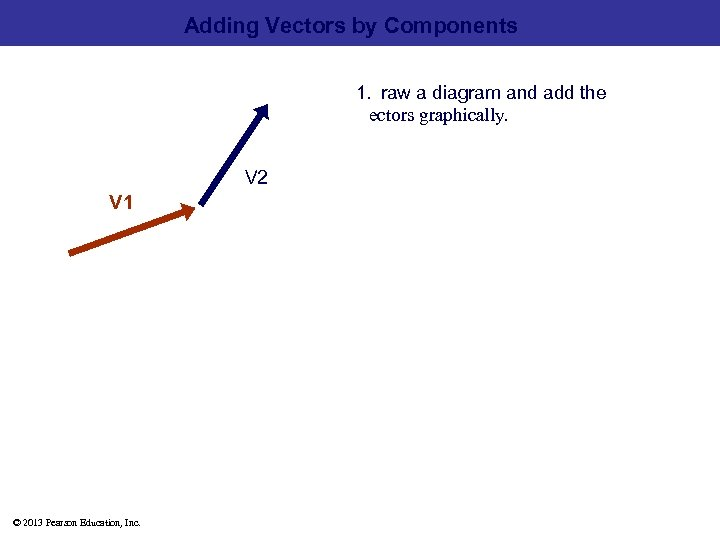Adding Vectors by Components 1.  raw a diagram and add the   ectors graphically. V 2 V 1 © 2013 Pearson Education, Inc.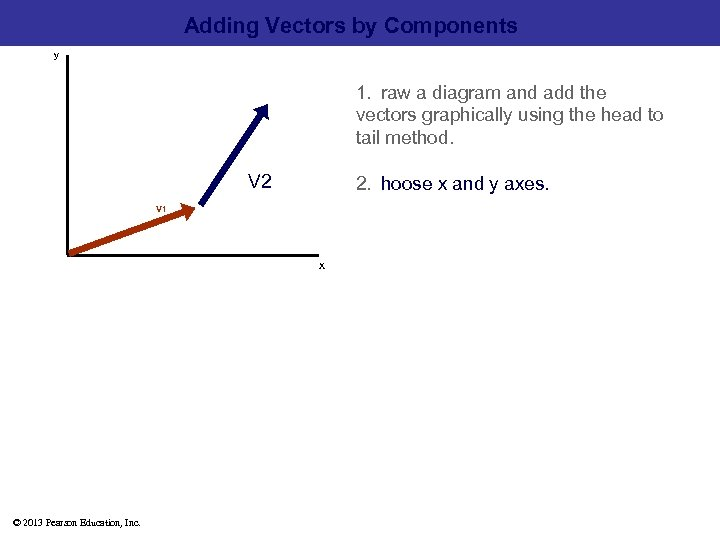Adding Vectors by Components y 1.  raw a diagram and add the    vectors graphically using the head to tail method. V 2 2.  hoose x and y axes. V 1 x © 2013 Pearson Education, Inc.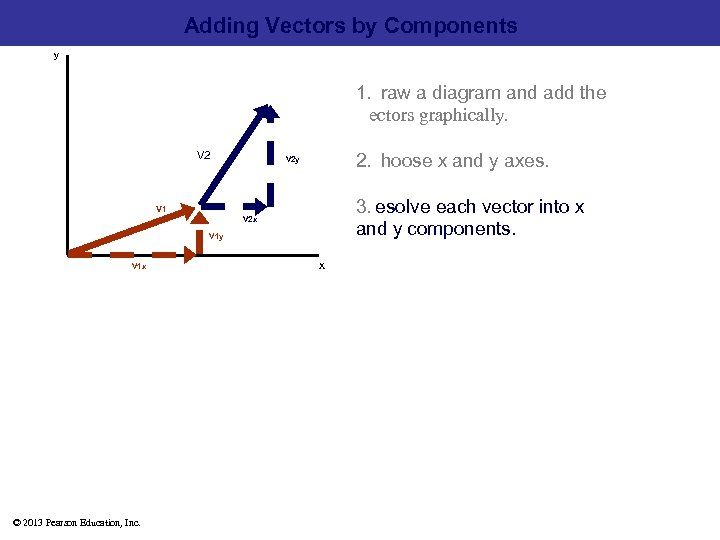Adding Vectors by Components y 1.  raw a diagram and add the   ectors graphically. V 2 2.  hoose x and y axes. V 2 y 3.  esolve each vector into x   and y components. V 1 V 2 x V 1 y V 1 x © 2013 Pearson Education, Inc. x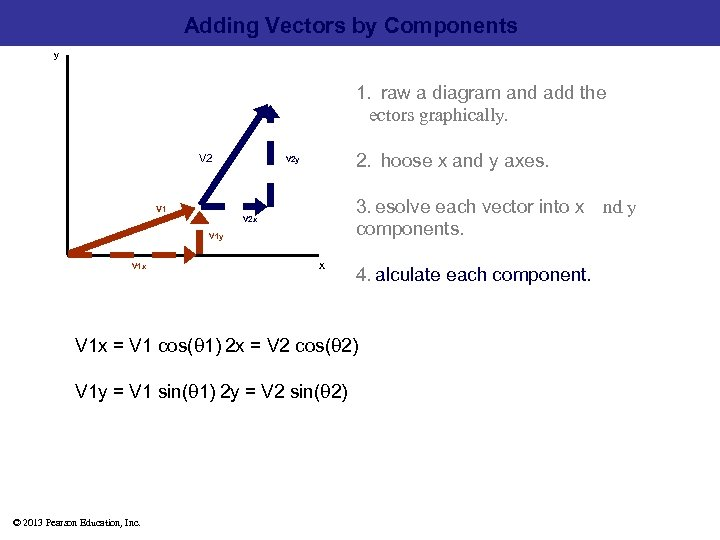Adding Vectors by Components y 1.  raw a diagram and add the   ectors graphically. V 2 2.  hoose x and y axes. V 2 y 3.  esolve each vector into x   nd y components. V 1 V 2 x V 1 y V 1 x x 4.  alculate each component. V 1 x = V 1 cos(θ 1)  2 x = V 2 cos(θ 2) V 1 y = V 1 sin(θ 1)  2 y = V 2 sin(θ 2) © 2013 Pearson Education, Inc.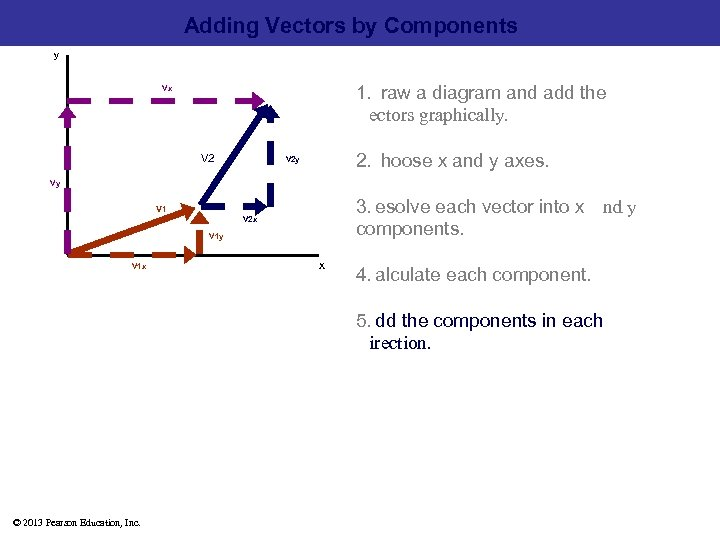Adding Vectors by Components y 1.  raw a diagram and add the   ectors graphically. Vx V 2 2.  hoose x and y axes. V 2 y Vy 3.  esolve each vector into x   nd y components. V 1 V 2 x V 1 y V 1 x x 4.  alculate each component. 5.  dd the components in each   irection. © 2013 Pearson Education, Inc.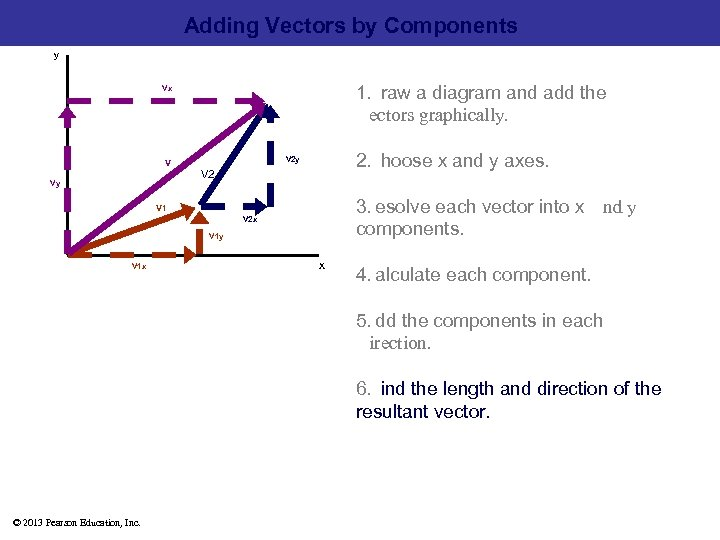Adding Vectors by Components y 1.  raw a diagram and add the   ectors graphically. Vx 2.  hoose x and y axes. V 2 y V V 2 Vy 3.  esolve each vector into x   nd y components. V 1 V 2 x V 1 y V 1 x x 4.  alculate each component. 5.  dd the components in each   irection. 6.  ind the length and direction of the resultant vector. © 2013 Pearson Education, Inc.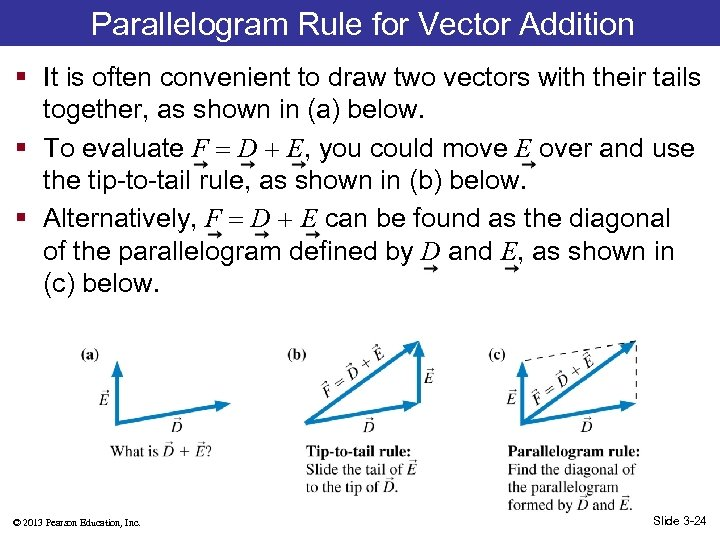Parallelogram Rule for Vector Addition § It is often convenient to draw two vectors with their tails together, as shown in (a) below. § To evaluate F D E, you could move E over and use the tip-to-tail rule, as shown in (b) below. § Alternatively, F D E can be found as the diagonal of the parallelogram defined by D and E, as shown in (c) below. © 2013 Pearson Education, Inc. Slide 3 -24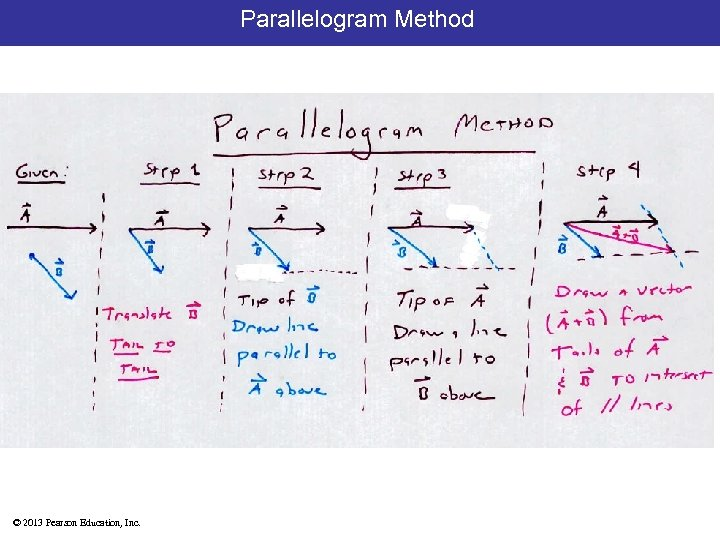Parallelogram Method © 2013 Pearson Education, Inc.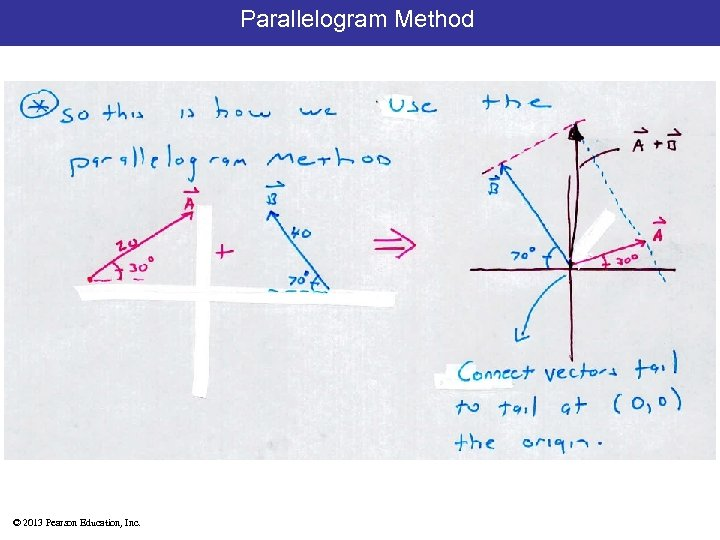Parallelogram Method © 2013 Pearson Education, Inc.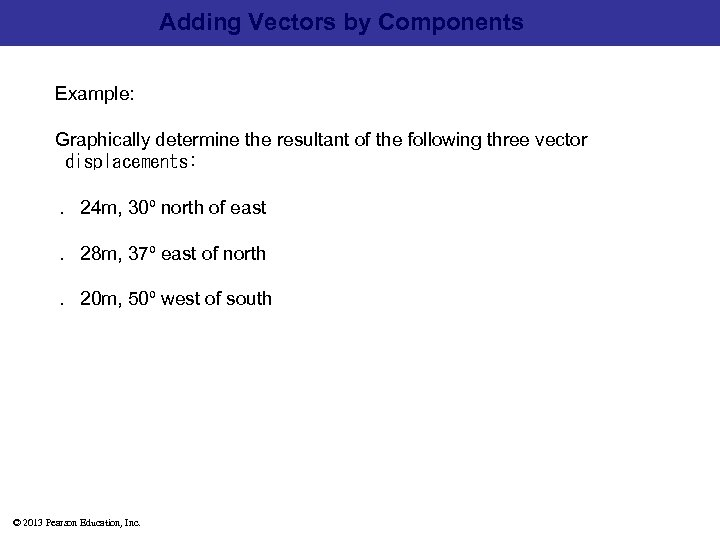Adding Vectors by Components Example: Graphically determine the resultant of the following three vector  displacements:  . 24 m, 30º north of east  . 28 m, 37º east of north  . 20 m, 50º west of south © 2013 Pearson Education, Inc.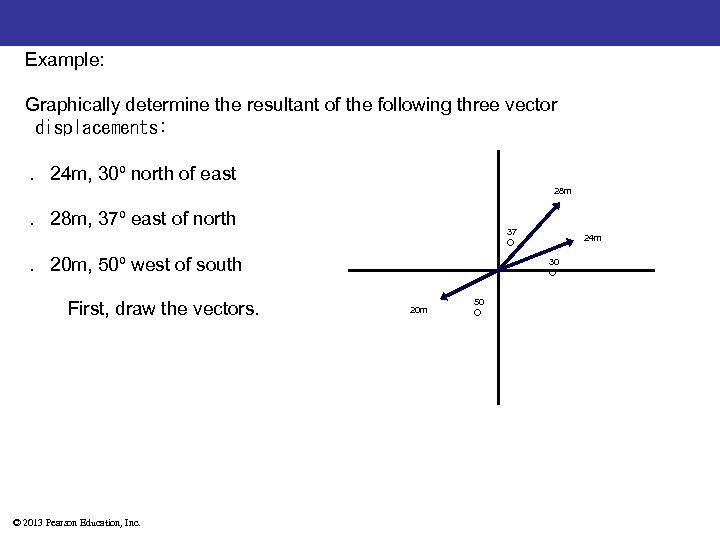Example: Graphically determine the resultant of the following three vector  displacements:  . 24 m, 30º north of east 28 m  . 28 m, 37º east of north 37 O  . 20 m, 50º west of south First, draw the vectors. © 2013 Pearson Education, Inc. 24 m 30 O 20 m 50 O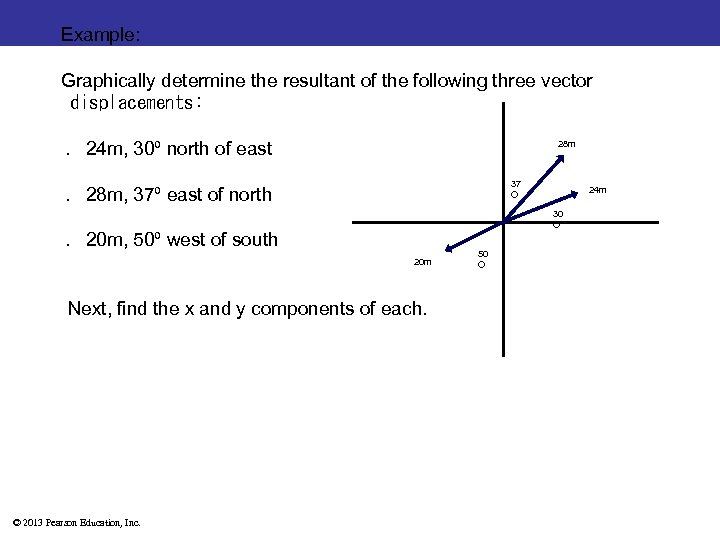Example: Graphically determine the resultant of the following three vector  displacements:  . 24 m, 30º north of east 28 m 37 O  . 28 m, 37º east of north 30 O  . 20 m, 50º west of south 20 m Next, find the x and y components of each. © 2013 Pearson Education, Inc. 24 m 50 OExample: Graphically determine the resultant of the following three vector  displacements:  . 24 m, 30º north of east 28 m 37 O  . 28 m, 37º east of north 30 O  . 20 m, 50º west of south 20 m Next, find the x and y components of each. © 2013 Pearson Education, Inc. 24 m 50 O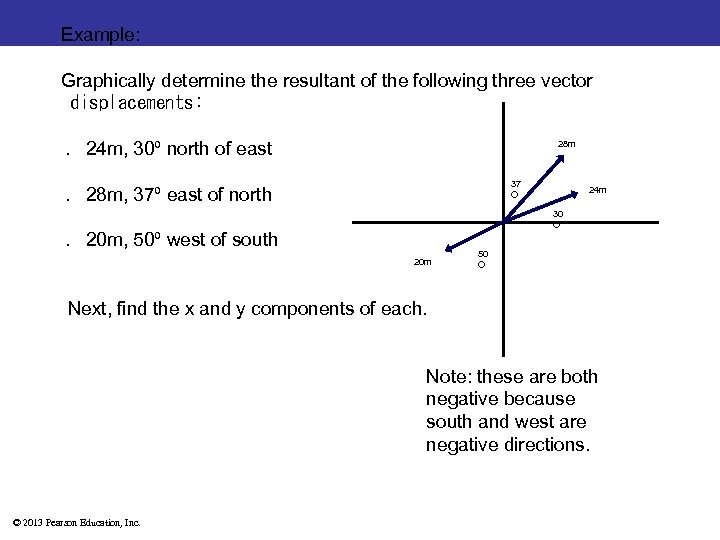Example: Graphically determine the resultant of the following three vector  displacements:  . 24 m, 30º north of east 28 m 37 O  . 28 m, 37º east of north 24 m 30 O  . 20 m, 50º west of south 20 m 50 O Next, find the x and y components of each. Note: these are both negative because south and west are negative directions. © 2013 Pearson Education, Inc.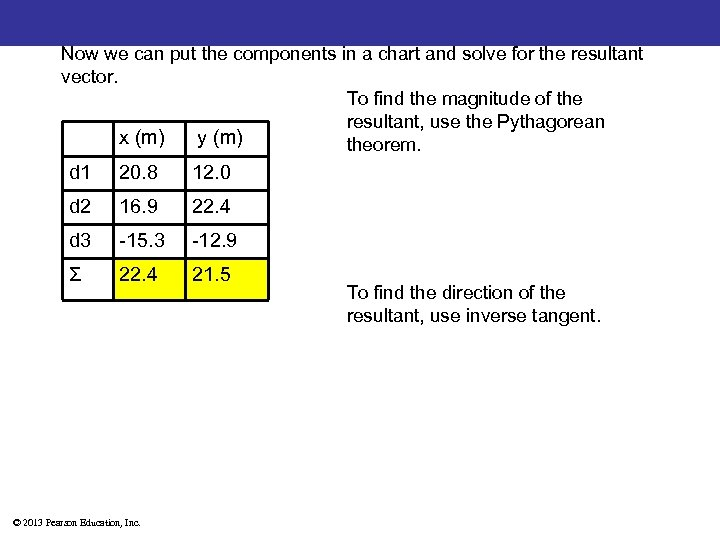Now we can put the components in a chart and solve for the resultant vector. To find the magnitude of the resultant, use the Pythagorean x (m) y (m) theorem. d 1 20. 8 12. 0 d 2 16. 9 22. 4 d 3 -15. 3 -12. 9 Ʃ 22. 4 21. 5 © 2013 Pearson Education, Inc. To find the direction of the resultant, use inverse tangent.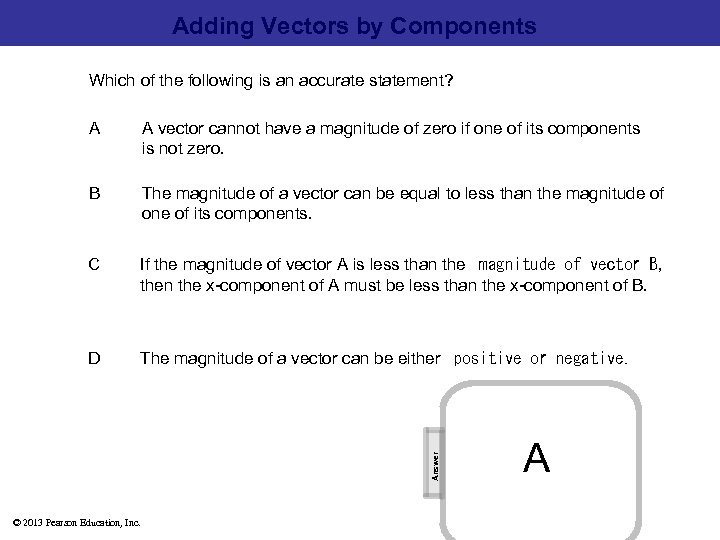Adding Vectors by Components Which of the following is an accurate statement? A vector cannot have a magnitude of zero if one of its components is not zero. B The magnitude of a vector can be equal to less than the magnitude of one of its components. C If the magnitude of vector A is less than the  magnitude of vector B, then the x-component of A must be less than the x-component of B. D The magnitude of a vector can be either  positive or negative. Answer A A © 2013 Pearson Education, Inc. [This object is a pull tab]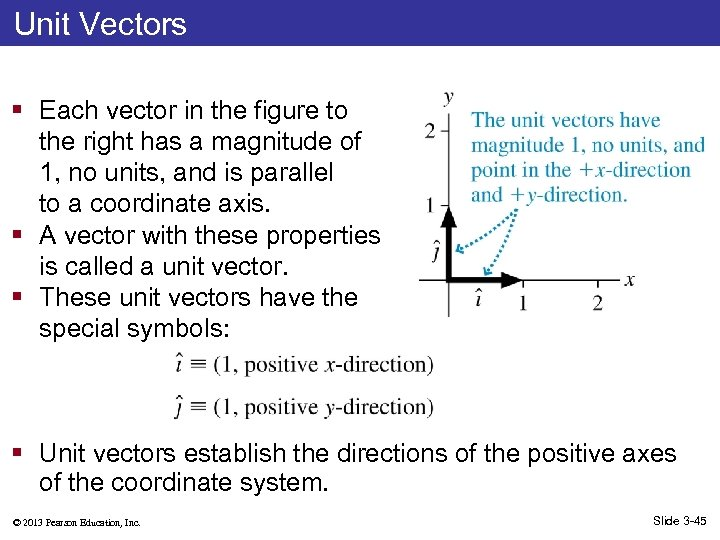Unit Vectors § Each vector in the figure to the right has a magnitude of 1, no units, and is parallel to a coordinate axis. § A vector with these properties is called a unit vector. § These unit vectors have the special symbols: § Unit vectors establish the directions of the positive axes of the coordinate system. © 2013 Pearson Education, Inc. Slide 3 -45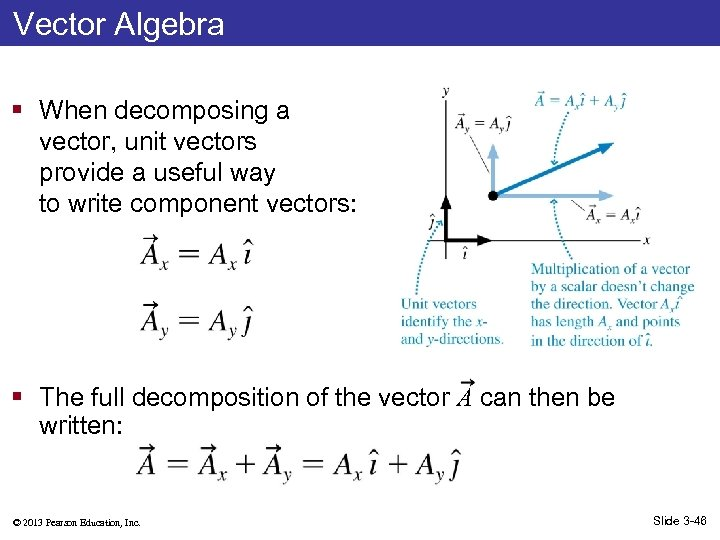Vector Algebra § When decomposing a vector, unit vectors provide a useful way to write component vectors: § The full decomposition of the vector A can then be written: © 2013 Pearson Education, Inc. Slide 3 -46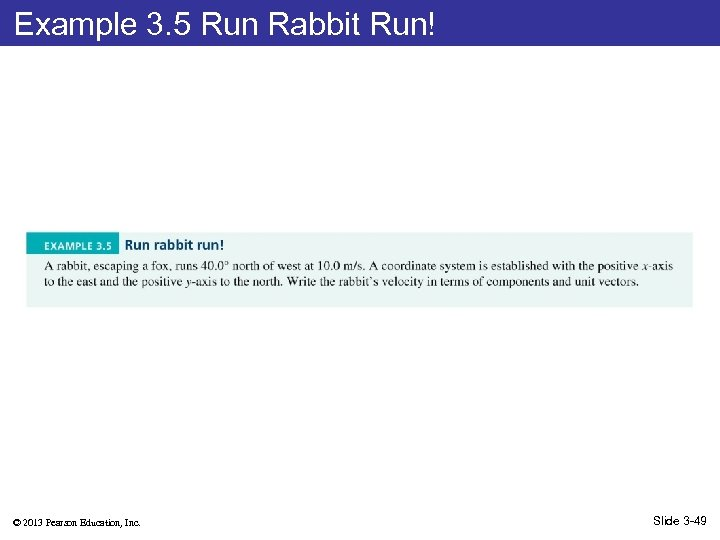Example 3. 5 Run Rabbit Run! © 2013 Pearson Education, Inc. Slide 3 -49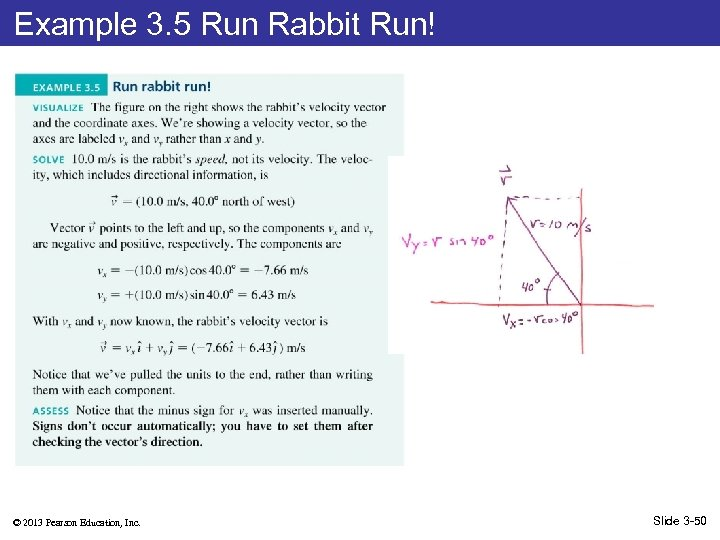Example 3. 5 Run Rabbit Run! © 2013 Pearson Education, Inc. Slide 3 -50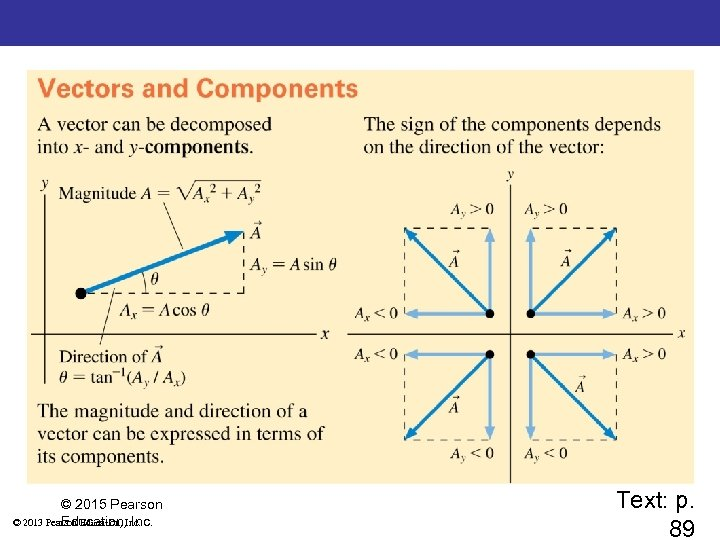Summary: Important Concepts © 2015 Pearson © 2013 Pearson Education, Inc. Text: p. 89